# Constrained and Non-Convex Optimization¶

## Unit 11, Lecture 2¶

Numerical Methods and Statistics

## Goals:¶

1. Learn how to perform non-convex optimization
2. Be able to bound optimization and root-finding problems
3. Add constraints to optimization and root-finding
4. Put all these skills together into one example
In :
%matplotlib inline
import numpy as np
import matplotlib.pyplot as plt
from math import sqrt, pi
import scipy.optimize


# Non-Convex Optimization¶

A highly-challenging, unsolvable problem. There is no general purpose non-convex optimization tool. Scipy uses a sort-of OK method called basin-hopping

In :
def non_convex(x):
return np.cos(14.5 * x - 0.3) + (x + 0.2) * x

x = np.linspace(-1,1, 100)
plt.plot(x, non_convex(x))
plt.show()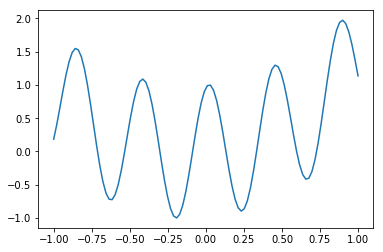In :
ret = scipy.optimize.basinhopping(non_convex, x0=1, niter=1000)
print(ret)

                        fun: -1.0008761844426555
lowest_optimization_result:       fun: -1.0008761844426555
hess_inv: array([[ 0.00471235]])
jac: array([  1.51991844e-06])
message: 'Optimization terminated successfully.'
nfev: 21
nit: 3
njev: 7
status: 0
success: True
x: array([-0.19506755])
message: ['requested number of basinhopping iterations completed successfully']
minimization_failures: 0
nfev: 23745
nit: 1000
njev: 7915
x: array([-0.19506755])


Notice you have to specify how long to try for. There is no way that basin hopping will know it's finished. Thus, try a few different iteration numbers and see if it changes.

In :
x = np.linspace(-1,1, 100)
plt.plot(x, non_convex(x))
plt.plot(ret.x, ret.fun, 'rp')
plt.show()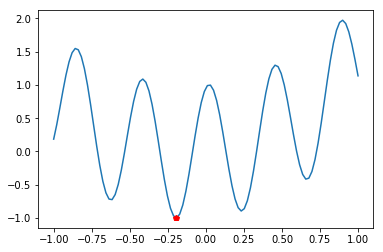# Basin-Hopping¶

Type: Non-convex wrapper to minimize

Discrete/Continuous: Continuous

Dimensions: N

Derivative: optional

Convex: not required

Python: basinhopping

Notes: Pass minimize arguments with a minimizer_kwargs=min_args, where min_args is a dictionary of arguments to the minimize function.

# Bounded Optimization¶

Often we want to restrict the domain of our objective function. This is done differently for root finding and minimization. There is no good method for bounded root finding in N-dimensions implemented in scipy.

# 1D Root finding with bounds¶

In :
scipy.optimize.brentq(lambda x: np.sin(x), a=-pi / 2., b = pi / 2)

Out:
0.0

# Brent's Method¶

Type: Bounded Root-finding

Discrete/Continuous: Continuous

Dimensions: 1

Derivative: No

Convex: yes

Python: brentq

Notes: a and b are two points on the function such that $b > a$ and the signs of $f(a)$ and $f(b)$ are opposite.

Let's try with $\sin(x)$

In :
scipy.optimize.brentq(lambda x: np.sin(x), a=0.25, b = 0.5)

---------------------------------------------------------------------------
ValueError                                Traceback (most recent call last)
<ipython-input-104-04eba40122a5> in <module>()
----> 1 scipy.optimize.brentq(lambda x: np.sin(x), a=0.25, b = 0.5)

/home/whitead/.local/lib/python3.4/site-packages/scipy/optimize/zeros.py in brentq(f, a, b, args, xtol, rtol, maxiter, full_output, disp)
436     if rtol < _rtol:
437         raise ValueError("rtol too small (%g < %g)" % (rtol, _rtol))
--> 438     r = _zeros._brentq(f,a,b,xtol,rtol,maxiter,args,full_output,disp)
439     return results_c(full_output, r)
440

ValueError: f(a) and f(b) must have different signs

Must have different signs!

In :
scipy.optimize.brentq(lambda x: np.sin(x), a=-0.5, b = 0.5)

Out:
0.0

# Reaction equilibrium example¶

Consider the reaction:

HA + H$_2$O $\rightarrow$ H$_3$O$^+$ + A$^{-1}$

Let's say that $k=32.5$. If we add 1 M of weak acid, then what is the equilibrium concentration?

$$\frac{[H+][A-]}{[HA]} = \frac{x\times x}{1 - x} = 32.5$$
$$\frac{x^2}{1-x} - 32.5 = 0$$
In :
k = 32.5

def Q(x):
return x ** 2 / (1 - x)
def obj(x):
return Q(x) - k

scipy.optimize.root(obj, x0=0)

Out:
    fjac: array([[ 1.]])
fun: array([ 0.])
message: 'The solution converged.'
nfev: 8
qtf: array([ -1.22213351e-12])
r: array([-0.99915843])
status: 1
success: True
x: array([-33.4709901])
In :
scipy.optimize.brentq(obj, a=0, b=1)

---------------------------------------------------------------------------
ZeroDivisionError                         Traceback (most recent call last)
<ipython-input-108-5694e2f82d78> in <module>()
----> 1 scipy.optimize.brentq(obj, a=0, b=1)

/home/whitead/.local/lib/python3.4/site-packages/scipy/optimize/zeros.py in brentq(f, a, b, args, xtol, rtol, maxiter, full_output, disp)
436     if rtol < _rtol:
437         raise ValueError("rtol too small (%g < %g)" % (rtol, _rtol))
--> 438     r = _zeros._brentq(f,a,b,xtol,rtol,maxiter,args,full_output,disp)
439     return results_c(full_output, r)
440

<ipython-input-107-e9d0accf3053> in obj(x)
4     return x ** 2 / (1 - x)
5 def obj(x):
----> 6     return Q(x) - k
7
8 scipy.optimize.root(obj, x0=0)

<ipython-input-107-e9d0accf3053> in Q(x)
2
3 def Q(x):
----> 4     return x ** 2 / (1 - x)
5 def obj(x):
6     return Q(x) - k

ZeroDivisionError: float division by zero
In :
scipy.optimize.brentq(obj, a=0, b=1 - 10**-9)

Out:
0.9709900992940665

So the equilibrium concentration of the acid is $1 - x = 0.03\textrm{M}$

# 1D Minimization with bounds¶

Let's try minimization with $\sin x$ bound on the interval $[-\pi, \pi]$

# Sequential Least SQuares Programming (SLSQP)¶

Type: Bounded or Bounded and Constrained Minimization

Discrete/Continuous: Continuous

Dimensions: N

Derivative: optional

Convex: yes

Python: minimize with bounds argument

Notes: bounds look like bounds=[(min_1, max_1), (min_2, max_2), ...]. One for each dimension

In :
scipy.optimize.minimize(lambda x: np.sin(x), x0=, bounds=[(-pi, pi)])

Out:
      fun: array([-1.])
hess_inv: <1x1 LbfgsInvHessProduct with dtype=float64>
jac: array([ 0.])
message: b'CONVERGENCE: NORM_OF_PROJECTED_GRADIENT_<=_PGTOL'
nfev: 12
nit: 4
status: 0
success: True
x: array([-1.57079634])

# 2D Minimization with bounds¶

Minimzie $\sin x\cos y$ to be on interval $[-\pi, \pi]$ for both $x$ and $y$

In :
scipy.optimize.minimize(lambda x: np.sin(x) * np.cos(x), x0=[0, 0], bounds=[(-pi, pi), (-pi, pi)])

Out:
      fun: -1.0
hess_inv: <2x2 LbfgsInvHessProduct with dtype=float64>
jac: array([ 0.,  0.])
message: b'CONVERGENCE: NORM_OF_PROJECTED_GRADIENT_<=_PGTOL'
nfev: 18
nit: 4
status: 0
success: True
x: array([-1.57079634,  0.        ])

# Bounded Minimization Example¶

The entropy of a discrete probability distribution is given by:

$$S = -\sum_Q p_i \ln p_i$$

where the sum is over the sample space of the distribution. Find the maximum entropy binomial distribution $p$ for $N = 5$

We're saying we know all but one parameter, $p$, so we'll find a $p$ such that entropy is maximized. This is a common technique in statistical learning, machine learning, and statistical mechanics. Much of my own research uses this idea of solving underdetermined problems by maximizing entropy.

In :
from scipy.special import comb
import numpy as np

#P(n)
def binomial(n, p, N):
return comb(N, n) * (1 - p) ** (N - n) * p**n

#Make sure this function works with numpy arrays
test = np.arange(3)
print(binomial(test, 0.2, 5))

[ 0.32768  0.4096   0.2048 ]

In :
#We need to use negative entropy, so it's a minimization problem
def negative_entropy(x):
p = x
N = 5
ps = binomial(np.arange(N + 1), p, N)
return np.sum(ps * np.log(ps))
negative_entropy(0.2)

Out:
-1.2430241623118781
In :
scipy.optimize.minimize(negative_entropy, x0=0.5, bounds=[(10**-9, 1 - 10**-9)])

Out:
      fun: -1.5236708720427921
hess_inv: <1x1 LbfgsInvHessProduct with dtype=float64>
jac: array([  4.44089210e-08])
message: b'CONVERGENCE: NORM_OF_PROJECTED_GRADIENT_<=_PGTOL'
nfev: 2
nit: 0
status: 0
success: True
x: array([ 0.5])
In :
np_negative_entropy = np.frompyfunc(negative_entropy, 1, 1)
x = np.linspace(0.01, 0.99, 25)
plt.plot(x, np_negative_entropy(x))
plt.xlabel('$p$')
plt.ylabel('S')
plt.show()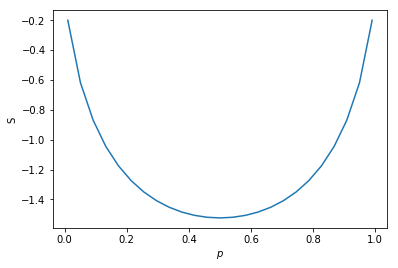So each $p$ value corresponds to an entire binomial distribution. Let's see how to plot each of them

In :
import matplotlib.cm as cm #import some colors

#need this to color based on entropy. It converts entropy to a number between 0 and 1
def entropy_to_01(x):
return (x - 0.1) / (1.5 - 0.1)

color_grad = cm.jet #my color scheme
n = np.linspace(0, 5, 6)
#plot the binomial for different ps, but colored by their entropy
for pi in np.linspace(0.01,0.99,25):
plt.plot(n, binomial(n, pi, 5), lw=1.5, color=color_grad(entropy_to_01(-negative_entropy(pi))))
plt.show()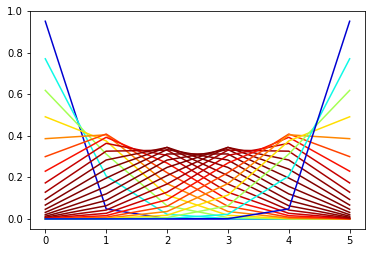# Constrained Minimization¶

Constraints are much more general than bounds. We can use arbitrary numbers of equality and inequality constraints that must be obeyed for a solution.

Consider $x$, $y$ which lie on the circle $x^2 + y^2 = 1$. Find the $x$, $y$ which is as close as possible to the point $(3,4)$.

In :
x = np.linspace(-1, 1, 100)

#make sure it's square
plt.figure(figsize=(6,6))

#have to plot the circle in two steps
plt.plot(x, np.sqrt(1 - x**2), color='blue')
plt.plot(x, -np.sqrt(1 - x**2), color='blue')

#now the point
plt.plot(3, 4, 'ro')

plt.xlim(-5, 5)
plt.ylim(-5, 5)

plt.show()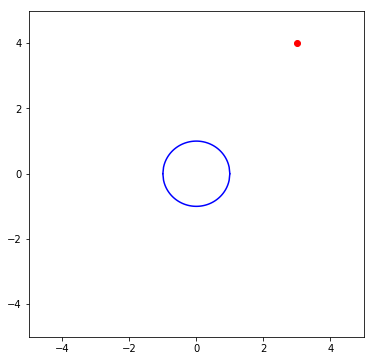In :
from math import sqrt

def dist(x1, x2):
return sqrt(np.sum((x1 - x2)**2))

x1 = np.array([1,5])
x2 = np.array([-2,4])
print(dist(x1, x2))

3.1622776601683795

In :
def circle(x):
return x ** 2 + x ** 2 - 1

print(circle([0, 1]))

0

In :
def obj(x):
return dist(x, np.array([3,4]))
print(obj(np.array([2,4])))

1.0

In :
my_constraints = {'type': 'eq', 'fun': circle}
result = scipy.optimize.minimize(obj, x0=[0,1], constraints=my_constraints)
print(result)

     fun: 3.9999999598768268
jac: array([-0.59999996, -0.80000007])
message: 'Optimization terminated successfully.'
nfev: 24
nit: 6
njev: 6
status: 0
success: True
x: array([ 0.60000024,  0.79999987])

In :
x = np.linspace(-1, 1, 100)

#make sure it's square
plt.figure(figsize=(6,6))

#plot the circle in 2 steps
plt.plot(x, np.sqrt(1 - x**2), color='blue')
plt.plot(x, -np.sqrt(1 - x**2), color='blue')
plt.plot(3, 4, 'ro')
plt.plot(result.x, result.x, 'go')
plt.xlim(-5, 5)
plt.ylim(-5, 5)

Out:
(-5, 5)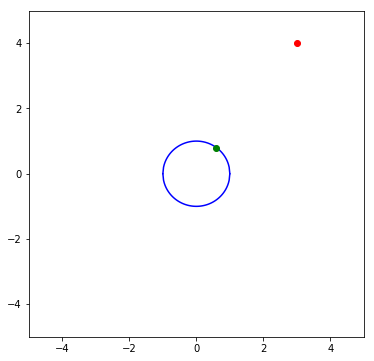# L-BFGS-B¶

Type: Constrained Minimization

Discrete/Continuous: Continuous

Dimensions: N

Derivative: optional

Convex: yes

Python: minimize with constraint arguments

# Python Dictionaries - Quick Refresher¶

In :
date = {'year': 2015, 'month':'October', 'day': 3}

print(date['year'])

2015

In :
print(date['month'])

October

In :
date['month'] = 'Footober'

print(date['month'])

Footober


Python dictionaries are used when you want to store multiple numed variables together.

In :
my_constraints = {'type': 'eq', 'fun': circle}

In :
my_constraints = {'type': 'ineq', 'fun': circle} #An inequality constraint


# Constraints¶

The constraints are like our work with the newton function, one side of the equation is always 0.

$$x^2 + y^2 = 1$$$$x^2 + y^2 - 1 = 0$$

Inequality means the quantity is always non-negative

$$x^2 - 2y > 4$$$$x^2 - 2y - 4 > 0$$
$$y < 34$$$$y - 34 < 0$$$$34 - y > 0$$

# Non-Convex Optimization with Constraints¶

Now that we're good with constraints, let's try to do the ultimate optimization problem: Non-convex with constraints

In :
from math import atan, sqrt, sin
x = np.linspace(-1, 1, 100)

#make sure it's square and make it polar
plt.figure(figsize=(8,8))
ax = plt.subplot(111, polar=True)
theta = np.linspace(0, 2 * pi, 500)
r = 1 + 0.3 * np.sin(7 * (theta + 4))
ax.plot(theta, r)
ax.plot(atan(2 / 1.), sqrt(2**2 + 1**2), 'bo')

ax.set_rmax(3)
plt.show()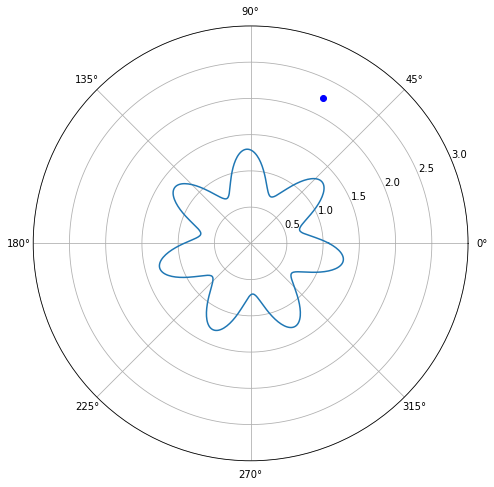We want to find a point on the flower that is as close as possible to the red dot. This is non-convex because there are many local minima.

In :
def circle_constraint(s):
x = s
y = s
r = sqrt(x**2 + y**2)
theta = atan(y / x)
return 1 + 0.3 * np.sin(7 * (theta + 4)) - r
print(circle_constraint(np.array([1,1])))

-0.152546225676

In :
def dist(x1, x2):
return sqrt(np.sum((x1 - x2)**2))

In :
def obj(x):
return dist(x, np.array([1,2]))
print(obj(np.array([0,1])))

1.4142135623730951


Basin-hopping is like a wrapper around one of the minimization techniques we've learned. So to communicate with the technique which basin-hopping is using, we have to wrap our constraint dictionary in another dictionary.

In :
my_constraints = {'type':'eq', 'fun':circle_constraint}
minimizer_args = {'constraints':my_constraints}
ret = scipy.optimize.basinhopping(obj, x0=[1,1], niter=1000, minimizer_kwargs=minimizer_args)

In :
print(ret)
print(circle_constraint(ret.x))
print(ret.x)

                        fun: 1.1131024276840382
lowest_optimization_result:      fun: 1.1131024276840382
jac: array([-0.09772378, -0.99521358])
message: 'Optimization terminated successfully.'
nfev: 20
nit: 5
njev: 5
status: 0
success: True
x: array([ 0.89122342,  0.89222535])
message: ['requested number of basinhopping iterations completed successfully']
minimization_failures: 0
nfev: 37362
nit: 1000
njev: 8748
x: array([ 0.89122342,  0.89222535])
-6.36809861732e-07
[ 0.89122342  0.89222535]

In :
x = np.linspace(-1, 1, 100)

#make sure it's square and make it polar
plt.figure(figsize=(8,8))
ax = plt.subplot(111, polar=True)
theta = np.linspace(0, 2 * pi, 1500)
r = 1 + 0.3 * np.sin(7 * (theta + 4))
ax.plot(theta, r)
ax.plot(atan(2. / 1.), sqrt(2**2 + 1**2), 'ro')
ax.plot(atan(ret.x / ret.x), sqrt(ret.x ** 2 + ret.x ** 2), 'o')

ax.set_rmax(3)
plt.show()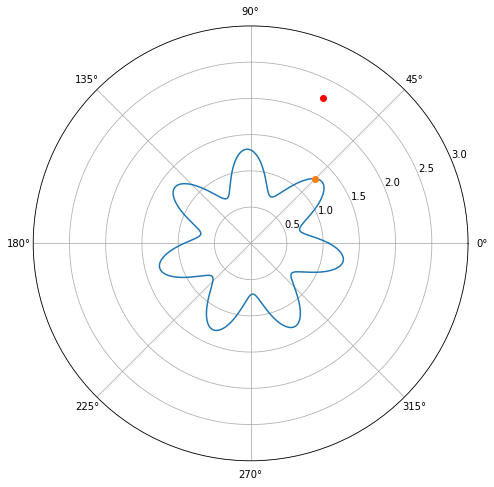# Basin-Hopping¶

Type: Non-convex wrapper to minimize

Discrete/Continuous: Continuous

Dimensions: N

Derivative: optional

Convex: not required

Python: basinhopping

Notes: Pass minimize arguments with a minimizer_kwargs=min_args, where min_args is a dictionary of arguments to the minimize function.